## PSEB 10th Class Maths Solutions Chapter 3 Pair of Linear Equations in Two Variables Ex 3.6

Punjab State Board PSEB 10th Class Maths Book Solutions Chapter 3 Pair of Linear Equations in Two Variables Ex 3.6 Textbook Exercise Questions and Answers.

## PSEB Solutions for Class 10 Maths Chapter 3 Pair of Linear Equations in Two Variables Ex 3.6

Question 1.
Solve the following pairs of equations by reducing them to a pair of linear equations:

(i) $$\frac{1}{2 x}+\frac{1}{3 y}$$ = 2
$$\frac{1}{3 x}+\frac{1}{2 y}=\frac{13}{6}$$

(ii) $$\frac{2}{\sqrt{x}}+\frac{3}{\sqrt{y}}$$ = 2
$$\frac{4}{\sqrt{x}}-\frac{9}{\sqrt{y}}$$ = -1

(iii) $$\frac{4}{x}$$ + 3y = 14
$$\frac{3}{x}$$ – 4y = 23

(iv) $$\frac{5}{x-1}+\frac{1}{y-2}$$ = 2
$$\frac{6}{x-1}-\frac{3}{y-2}$$ = 1

(v) $$\frac{7 x-2 y}{x y}$$ = 5
$$\frac{8 x+7 y}{x y}$$ = 15

(vi) 6x + 3y = 6xy
2x + 4y = 5xy

(vii) $$\frac{10}{x+y}+\frac{2}{x-y}$$ = 4
$$\frac{15}{x+y}-\frac{5}{x-y}$$ = -2

(viii) $$\frac{1}{3 x+y}+\frac{1}{3 x-y}=\frac{3}{4}$$
$$\frac{1}{2(3 x+y)}-\frac{1}{2(3 x-y)}=\frac{-1}{8}$$Solution:
(i) Given pair of linear equations are;
$$\frac{1}{2 x}+\frac{1}{3 y}$$ = 2
$$\frac{1}{3 x}+\frac{1}{2 y}=\frac{13}{6}$$
putting $$\frac{1}{x}$$ = u and $$\frac{1}{x}$$ = v, then equations reduces to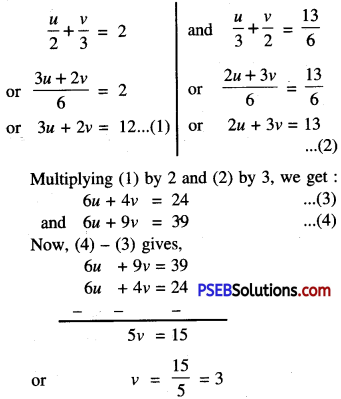Substitute this value of v in (1), we get:
3u + 2 (3) = 12
or 3u + 6 = 12
or 3u = 12 – 6 = 6
or u = $$\frac{6}{3}$$ = 2
But $$\frac{1}{x}$$ = u
or x = $$\frac{1}{u}$$
∴ x = $$\frac{1}{2}$$

and $$\frac{1}{y}$$ = v
y = $$\frac{1}{v}$$
or y=—$$\frac{1}{3}$$
Hence x = $$\frac{1}{2}$$ and y = $$\frac{1}{3}$$(ii) Given pair of linear equation are
$$\frac{2}{\sqrt{x}}+\frac{3}{\sqrt{y}}$$ = 2
$$\frac{4}{\sqrt{x}}-\frac{9}{\sqrt{y}}$$ = -1
Putting $$\frac{1}{\sqrt{x}}$$ = u and $$\frac{1}{\sqrt{y}}$$ = v then equations reduces to
2u + 3v = 2 ……………(1)
and 4u – 9v = -1 ……………(2)
Multiplying (1) by 2, we get:
4u + 6v = 4
Now, (2) – (3) gives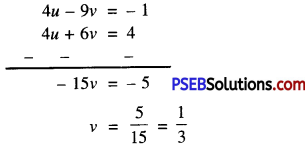Substitute this value of v in (1), we get:
2u + 3($$\frac{1}{3}$$) = 2
or 2u + 1 = 2
or 2u = 2 – 1 = 1
or u = $$\frac{1}{2}$$

But $$\frac{1}{\sqrt{x}}$$ = u
or ($$\frac{1}{\sqrt{x}}$$)2 = u2
or $$\frac{1}{x}$$ = u2
or $$\frac{1}{x}$$ = ($$\frac{1}{2}$$)2
or x = 4

and $$\frac{1}{\sqrt{y}}$$ = v
or ($$\frac{1}{\sqrt{y}}$$)2 = v2
or $$\frac{1}{y}$$ = v2
or $$\frac{1}{y}$$ = ($$\frac{1}{3}$$)2
or y = 9
Hence x = 4 and y = 9.(iii) Given pair of linear equations are
$$\frac{4}{x}$$ + 3y = 14 and $$\frac{3}{x}$$ – 4y = 23
putting $$\frac{1}{y}$$ = v then equations reduces to
4v + 3y = 14 ………..(1)
and 3v – 4y = 23 ……………(2)
Multiplying (1) by 3 and (2) by 4, we get:
12v + 9y = 42 ………..(3)
and 12v – 16y = 92 ……………..(4)
Now, (4) – (3) gives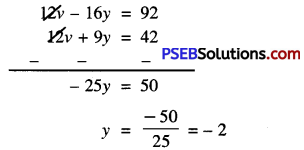Substitute this value of y in (1), we get:
4v + 3(-2) = 14
or 4v – 6 = 14
or 4v = 14 + 6 = 20
or v = $$\frac{20}{4}$$ = 5
But $$\frac{1}{x}$$ = v
x = $$\frac{1}{v}$$ = $$\frac{1}{5}$$
Hence x = $$\frac{1}{5}$$ and y = -2.(iv) Given pair of linear equation are
$$\frac{5}{x-1}+\frac{1}{y-2}$$ = 2 and $$\frac{6}{x-1}-\frac{3}{y-2}$$ = 12
Putting $$\frac{1}{x-1}$$ = u and $$\frac{1}{y-2}$$ = v then equations reduces to
5u + v = 2 ……………(1)
and 6u – 3v = 1 ……………(2)
Multiplying (1) by 3, we get:
15u + 3v = 6 ………..(3)
Now, (3) + (2) gives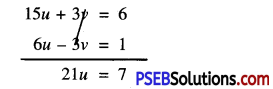u = $$\frac{7}{21}=\frac{1}{3}$$
Substitute this value of u in (1), we get:
5 × $$\frac{1}{3}$$ + v = 2
or v = 2 – $$\frac{5}{3}$$ = $$\frac{6-5}{3}$$
or v = $$\frac{1}{3}$$

But $$\frac{1}{x-1}$$ = u
or $$\frac{1}{x-1}$$ = $$\frac{1}{3}$$
or x – 1 = 3
or x = 3 + 1
or x = 4

and $$\frac{1}{y-2}$$ = v
or $$\frac{1}{y-2}$$ = $$\frac{1}{3}$$
or y – 2 = 3
or y = 3 + 2
or y = 5
Hence x = 4 or y = 5.(v) Given pair of linear equation are
$$\frac{7 x-2 y}{x y}$$ = 5
or $$\frac{7 x}{x y}-\frac{2 y}{x y}$$ = 5
or $$\frac{7}{y}-\frac{2}{x}$$ = 5
or $$-\frac{2}{x}+\frac{7}{y}$$ = 5

and $$\frac{8 x+7 y}{x y}$$ = 15
or $$\frac{8 x}{x y}+\frac{7 y}{x y}$$ = 15
or $$\frac{8}{y}+\frac{7}{x}$$ = 15
or $$\frac{7}{x}+\frac{8}{y}$$ = 15

Putting $$\frac{1}{x}$$ = u and $$\frac{1}{y}$$ = v, then equations reduces to
-2u + 7v = 5 ………….(1)
and 7u + 8v = 15 ………….(2)
Multiplying (1) by 7 and (2) by 2, we get:
-14v + 49u = 35
and 14v + 16u = 30
Now, (3) + (4) gives,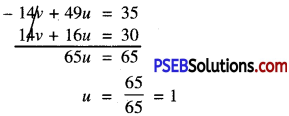Substitute thuis value of u in (1), we get:
-2(1) + 7v = 5
or 7v = 5 + 2
or 7v = 7
or v = $$\frac{7}{7}$$ = 1

But $$\frac{1}{x}$$ = u
or x = $$\frac{1}{u}$$
or x = $$\frac{1}{1}$$
or x = 1

and $$\frac{1}{y}$$ = v
or y = $$\frac{1}{v}$$
or y = $$\frac{1}{1}$$
or y = 1
Hence x = 1 and y = 1.

(vi) Given pair of linear equations are
6x + 3y = 6xy
or $$\frac{6 x+3 y}{x y}=\frac{6 x y}{x y}$$
or $$\frac{6}{y}+\frac{3}{x}=6$$
or $$3\left[\frac{1}{x}+\frac{2}{y}\right]=6$$
or $$\frac{1}{x}+\frac{2}{y}=2$$

and 2x + 4y = 5xy
or $$\frac{2 x+4 y}{x y}=\frac{5 x y}{x y}$$
or $$\frac{2}{y}+\frac{4}{x}=5$$
or $$\frac{4}{x}+\frac{2}{y}=5$$
Putting $$\frac{1}{x}$$ = u and $$\frac{1}{y}$$ = v, then equations reduces to
u + 2v = 2 ……………(1)
and 4u + 2v = 5 ……….(2)
Now, (2) – (1) gives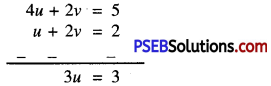or u = $$\frac{3}{3}$$ = 1
Substitute this value of u in (1), we get:
1 + 2v = 2
or 2v = 2 – 1
or v = $$\frac{1}{2}$$

But $$\frac{1}{x}$$ = u
or $$\frac{1}{x}$$ = 1
or x = 1

and $$\frac{1}{y}$$ = v
or $$\frac{1}{y}$$ = $$\frac{1}{2}$$
or y = 2
Hence x = 1 and y = 2.(vii) Given pair of linear equations are
$$\frac{10}{x+y}+\frac{2}{x-y}$$ = 4
$$\frac{15}{x+y}-\frac{5}{x-y}$$ = -2
Putting $$\frac{1}{x+y}$$ = u and $$\frac{1}{x-y}$$ = v, then equations reduces to
10u + 2v = 4 or
5u + v = 2 …………(1)
15u – 5v = -2 ………….(2)
Multiplying (1) by 5, we get
25u + 5v = 10 ………….(3)
Now, (3) + (2) gives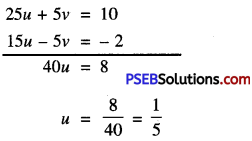Substitute this value of u in (1), we get:
5($$\frac{1}{5}$$) + v = 2
or 1 + v = 2
or v = 1
But $$\frac{1}{x+y}$$ = u
or $$\frac{1}{x+y}$$ = $$\frac{1}{5}$$
or x + y = 5 ……….(4)
and $$\frac{1}{x-y}$$ = v
or $$\frac{1}{x-y}$$ = 1
or x – y = 1 ………..(5)
Now, (4) + (5) gives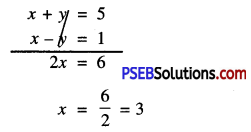Substitute this value of x in (4), we get:
3 + y = 5
y = 5 – 3 = 2
Hence x = 3 and y = 2.

(viii) Given pair of linear equations are
$$\frac{1}{3 x+y}+\frac{1}{3 x-y}=\frac{3}{4}$$ and $$\frac{1}{2(3 x+y)}-\frac{1}{2(3 x-y)}=\frac{-1}{8}$$
Putting $$\frac{1}{3 x+y}$$ = u and $$\frac{1}{3 x-y}$$ = y, then Equations reduces to
u + v = $$\frac{3}{4}$$
or 4u + 4v = 3
or 4u + 4v = 3 ………….(1)

and $$\frac{u}{2}-\frac{v}{2}=\frac{-1}{8}$$
or u – v = $$\frac{-1}{4}$$
or 4u – 4v = -1 ………………(2)
Now, (1) + (2) gives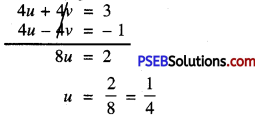Substitute this value of u in (1), we get:
4($$\frac{1}{4}$$) + 4v = 3
or 4v = 2
or v = $$\frac{2}{4}=\frac{1}{2}$$
But $$\frac{1}{3 x+y}$$ = $$\frac{1}{4}$$
or 3x + y = 4 …………(3)
and $$\frac{1}{3 x-y}$$ = $$\frac{1}{2}$$
or 3x – y = 2 …………….(4)
Now, (3) + (4) gives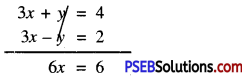x = 1
Substitute this value of x in (3), we get:
3(1) + y = 4
or 3 + y = 4
or y = 4 – 3 = 1
Hence, x = 1 and y = 1.Question 2.
Formulate the following problems as a pair of equations, and hence find their solutions.
(i) Ritu can row downstream 20 km in 2 hours, and upstream 4 km In 2 hours. Find her speed of rowing in still water and the speed of the current.

(ii) 2 women and 5 men can together finish an embroidery work in 4 days, while 3 women and 6 men can finish it in 3 days. Find the time taken by 1 woman alone to finish the work, and also that taken by 1 man alone.

(iii) Roohi travels 300 km to her home party by train and partly by bus. She takes 4 hours if she travels 60 km by train and the remaining by bus. If she travels 100 km by train and the remaining by bus, she takes 10 minutes longer. Find the speed of the train and the bus separately.Solution:
(i) Let the speed of Ritu in still water = x km/hour
and the speed of current = y km/hour
∴ speed in upstream = (x – y) km/hour
and speed in downstream = (x + y) km/hour
Distance covered by Ritu in downstream in 2 hours = Speed × Time
= (x + y) × 2 km
According to 1st condition
2(x + y) = 20
x + y = 10 ……………..(1)
Distance covered by Rim in upstream in 2 hours
= Speed × Time
= 2(x – y)km
According to 2nd condition,
2(x – y) =4
x – y = 2 ……………….(2)
Now, (1) + (2) gives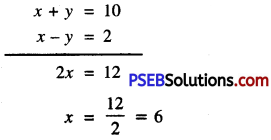Substitute this value of x in (1), we get:
6 + y = 10
y = 10 – 6 = 4
Hence, Ritu’s speed in still water = 6 km/hour
and speed of current = 4 km/hour.

(ii) Let one woman can fmish the work = x days
One man can finish the work = y days
then one woman’s one day’s work = $$\frac{1}{x}$$
One man’s one day’s work = $$\frac{1}{y}$$
According to 1st condition,
$$\frac{2}{x}+\frac{5}{y}=\frac{1}{4}$$ ……………(1)
According to 2nd equation
$$\frac{3}{x}+\frac{6}{y}=\frac{1}{3}$$ ……………(2)
Putting $$\frac{1}{x}$$ = u and $$\frac{1}{y}$$ = v, then equations (1) and (2) reduces to
2u + 5v = $$\frac{1}{4}$$
8u + 20v = 1 …………….(3)
and 3u + 6v = $$\frac{1}{3}$$
9u + 18v = 1 ……………..(4)
Multiplying (3) by 9 and (4) by 8, we get:
72u + 180v = 9 ……………..(5)
and 72u + 144v = 8 …………..(6)
Now, (5) – (6) gives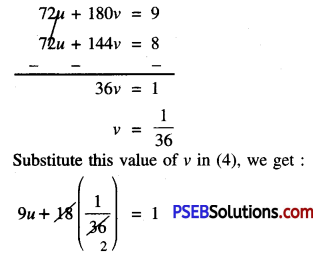or 9u + $$\frac{1}{2}$$ = 1
or 9u = 1 – $$\frac{1}{2}$$ = $$\frac{2-1}{2}$$
or 9u = $$\frac{1}{2}$$
or u = $$\frac{1}{2 \times 9}=\frac{1}{18}$$
But $$\frac{1}{x}$$ = u
or $$\frac{1}{x}$$ = $$\frac{1}{18}$$
or x = 18
and $$\frac{1}{y}$$ = v
$$\frac{1}{y}$$ = $$\frac{1}{36}$$
or y = 36
Hence, one woman and one man alone can finish work in 18 days and 36 days respectively.(iii) Let speed of train = x km/hour
and speed of bus = y km/hour
Total distance = 300 km
Case I:
Time taken by train to cover 60 km = $$\frac{\text { Distance }}{\text { Speed }}$$
= $$\frac{60}{x}$$ hours
Time taken by bus to cover 240 km = (300 – 60) = $$\frac{240}{y}$$ hours
Total time = $$\left(\frac{60}{x}+\frac{240}{y}\right)$$ hours
According to 1st condition,
$$\frac{60}{x}+\frac{240}{y}$$ = 4
$$\frac{15}{x}+\frac{60}{y}$$ = 1 ……………….(1)

Case II:
Time taken by train to cover 100 km = $$\frac{100}{x}$$ hours
Time taken by bus to cover 200 km = 300 – 100 = $$\frac{200}{y}$$ hours
∴ Total time = $$\left(\frac{100}{x}+\frac{200}{y}\right)$$ hours
According to 2nd condition,
$$\left(\frac{100}{x}+\frac{200}{y}\right)$$ = 4 hours 10 minutes
or $$\left(\frac{100}{x}+\frac{200}{y}\right)$$ = $$\frac{25}{6}$$
$$\left(\frac{24}{x}+\frac{48}{y}\right)$$ = 1 ……….(2)
Putting $$\frac{1}{x}$$ = u and $$\frac{1}{y}$$ = v in equations
(1) and (2) then equations reduces to
15u + 60v = 1
and 24u + 48v = 1
15u + 60v – 1 = 0
24u + 48v – 1 = 0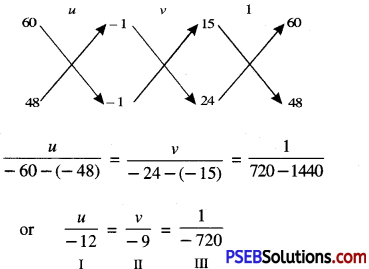From I and III, we get:
$$\frac{u}{-12}=\frac{1}{-720}$$
⇒ u = $$\frac{12}{720}=\frac{1}{60}$$
From II and III, we get:
$$\frac{v}{-9}=\frac{1}{-720}$$
⇒ v = $$\frac{9}{720}=\frac{1}{80}$$
But $$\frac{1}{x}$$ = u
or $$\frac{1}{x}$$ = $$\frac{1}{u}$$
or x = 60
and $$\frac{1}{y}$$ = v
or $$\frac{1}{y}$$ = $$\frac{1}{80}$$
or y = 80
Hence, speed of train and bus are 60 km/hour and 80 km/hour respectively.

## PSEB 10th Class Maths Solutions Chapter 3 Pair of Linear Equations in Two Variables Ex 3.4

Punjab State Board PSEB 10th Class Maths Book Solutions Chapter 3 Pair of Linear Equations in Two Variables Ex 3.4 Textbook Exercise Questions and Answers.

## PSEB Solutions for Class 10 Maths Chapter 3 Pair of Linear Equations in Two Variables Ex 3.4

Question 1.
Solve the following pair of equations by the elimination method and the substitution method.
(i) x + y = 5 and 2x – 3y = 4
(ii) 3x + 4y = 10 and 2x – 2y = 2
(iii) 3x – 5y – 4= 0 and 9x = 2y + 7
(iv) $$\frac{x}{2}+\frac{2 y}{3}$$ and x – $$\frac{y}{3}$$ = 3
Solution:
(i) Given pair of linear equations
x + y = 5 …………..(1)
and 2x – 3y = 4 ………….(2)
Elimination Method
Multiplying (1) by 2, we get:
2x + 2y = 10 …………..(3)

Now, (3) – (2) gives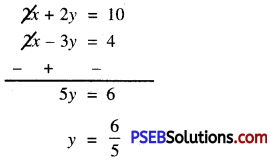Substitute this value of y in (1), we get:
x + $$\frac{6}{5}$$ = 5
or x = 5 – $$\frac{6}{5}$$
= $$\frac{25-6}{5}$$ = $$\frac{19}{5}$$
Hence, x = $$\frac{19}{5}$$ and $$\frac{6}{5}$$Substitution Method:

From(2), 2x =4 + 3y
or x = $$\frac{4+3 y}{2}$$ ……………..(4)
Substitute this value of x in (I), we get :
$$\frac{4+3 y}{2}$$ + y = 5
Or $$\frac{4+3 y+2 y}{2}$$
Or 4 + 5y = 10
Or 5y = 10 – 4 = 6
Or y = $$\frac{6}{5}$$
Substitute this value of y in (4). we get:
x = $$\frac{4+\left(3 \times \frac{6}{5}\right)}{2}=\frac{4+\frac{18}{5}}{2}$$
= $$\frac{20+18}{5 \times 2}=\frac{38}{5 \times 2}$$ = $$\frac{19}{5}$$
Hence x = $$\frac{19}{5}$$ and y = $$\frac{6}{5}$$

(ii) Given pair of linear equation is :
3x + 4y = 10 …………….(1)
and 2x – 2y = 2 …………………(2)
Elimination Method
Multiplying equation (2) by 2, we get:
4x – 4y = 4 ……………(3)
Now, (3) + (1) gives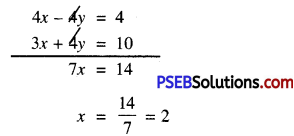Substitute this value of x in (1), we get:
3(2) + 4y = 10
or 6 + 4y = 10
or 4y = 10 – 6
or 4y = 4
or y = $$\frac{4}{4}$$ = 1
Hence x = 2 and y = 1.Substitution method:

From (2),
2x = 2 + 2y
or x = y + 1 ………..(3)
Substitute this value of x in (1), we get :
3(y + 1) +4y = 10
or 3y + 3 + 4y = 10
or 7y = 10 – 3
or 7y = 7
or y = 1
Substitute this value of y in (3), we get :
x = 1 + 1 = 2
Hence, x = 2 and y = 1.

(iii) Given pair of linear equation is :
3x – 5y – 4 = 0 ……..(1)
and 9x = 2y + 7
or 9x – 2y – 7 = 0
Elimination Method:

Multiplying (1) by 3, we get:
9x – 15y – 12 = 0 ……………(3)
Now, (3) – (2) gives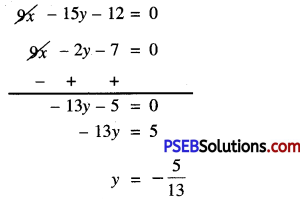Substitute this value of y in (1), we get:
3x – 5(-$$\frac{5}{13}$$) – 4 = 0
or 3x + $$\frac{25}{13}$$ – 4 = 0
or 3x = 4 – $$\frac{25}{13}$$
or 3x = $$\frac{52-25}{13}$$ = $$\frac{27}{13}$$
or x = $$\frac{27}{13} \times \frac{1}{3}$$
= $$\frac{9}{13}$$
Hence, x = $$\frac{9}{13}$$ and y = – $$\frac{5}{13}$$.Substitution Method:

From(2), x = $$\frac{2 y+7}{9}$$ …………..(4)
Substitute this value of x in (1), we get:
3$$\frac{2 y+7}{9}$$ – 5y – 4 = 0
or $$\frac{2 y+7-15 y-12}{3}$$ = 0
or – 13y – 5 = 0
or -13y = 5
or y = $$-\frac{5}{13}$$
Substitute this value of y in (4), we get: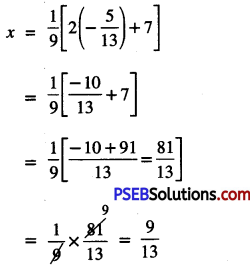Hence x = $$\frac{9}{13}$$ and y = –$$\frac{5}{13}$$

(iv) Given pair of linear equation is:
$$\frac{x}{2}+\frac{2 y}{3}$$ = -1
or $$\frac{3 x+4 y}{6}$$ = -1
or 3x + 4y = -6 ……………(1)
x – $$\frac{y}{3}$$ = 3
or $$\frac{3 x- y}{3}$$ = 3
or 3x – y = 9 ……………(2)

Elimination Method: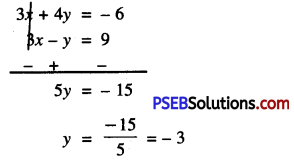Substitute this value of y in (1), we get :
3x + 4(-3) = -6
or 3x – 12 = -6
or 3x = -6 + 12
or 3x = 6
x = $$\frac{6}{3}$$ = 2
Hence x = 2, y = – 3.Substitution Method:

From (2), y = 3x – 9 …………..(4)
Substitute this value of y in (1), we get :
3x + 4(3x – 9) = -6
or 3x + 12x – 36 = -6
or 15x = -6 + 36
or 15x = 30
or x = $$\frac{30}{15}$$ = 2
Substitute this value of x in (4), we get :
y = 3 (2) – 9
= 6 – 9 = -3
Hence x = 2, y = -3.

Question 2.
Form the pair of linear equations in the following problems, and find their solutions (if they exist) by the elimination method:
(i) If we add 1 to the numerator and subtract 1 from the denominator, a fraction reduces to 1. It becomes if
we only add 1 to the denominator. What ¡s the fraction?

(ii) Five years ago, Nun was thrice as old as Sonu. Ten years later, Nun will be twice as old as Sonu. How old are Nun
and Sonti?

(iii) The sum of the digits of a two-digit nunaber ¡s 9. Also, nine times this number is twice the number obtained by reversing the order of the number. Find the number.

(iv) Meena went to a bank to withdraw ₹ 2000. She asked the cashier to give her ₹ 50 and ₹ 100 notes only. Meena got ₹ 25 notes in all. Find how many notes of ₹ 50 and ₹ 100 she received.

(v) A lending library has a fixed charge for the first three days and an additional charge for each day thereafter. Saritha paid ₹ 27 for a book kept for seven days, while Susy paid ₹ 21 for the book she kept for five days. Find the fixed charge and the charge for each extra day.Solution:
(i) Let numerator of fraction = x
Denominator of fraction = y
Required fraction = $$\frac{x}{y}$$
According to 1st condition,
$$\frac{x+1}{y-1}$$ = 1
or x + 1 = y – 1
or x – y + 2 = 0 …………….(1)
According to 2nd condition,
$$\frac{x}{y+1}=\frac{1}{2}$$
or 2x = y + 1
or 2x – y – 1 = 0 ……………..(2)
Now, (2) – (1) gives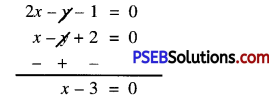or x = 3
Substitute this value of x in (2), we get :
2 × 3 – y – 1 = 0
or 6 – y – 1 = 0
or 5 – y = 0
or y = 5
Hence, required fraction is $$\frac{3}{5}$$.

(ii) Let Nun’s present age = x years
Sonus present age = y years
Five years ago
Nun’s age = (x – 5) years
Sonus age = (y – 5) years
According to 1st condition,
x – 5 = 3(y – 5)
or x – 5 = 3y – 15
or x – 3y + 10 = 0 …………….(1)
Ten years later
Nun’s age = (x + 10) years
Sonu’s age = (y + 10) years
According to 2nd condition,
x + 10 = 2 (y + 10)
or x + 10 = 2y + 20
or x – 2y – 10 = 0
Now, (1) – (2) gives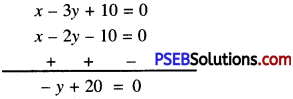or -y = -20
or y = 20
Substitute this value of y in (2), we get:
x – 2(20) – 10 = 0
or x – 40 – 10 = 0
or x = 50
Hence, Nun’s present age = 50 years
Sonu’s present age = 20 years.(iii) Let unit’s digit = x
Ten’s digit = y
∴ Required Number = 10y + x
According to 1st condition,
x + y = 9 …………..(1)
On reversing
Unit’s digit = y
Ten’s digit = x
∴ Number = 10x + y
According to 2nd condition,
9[10y + x] = 2[10x + y]
or 90y + 9x = 20x + 2y
or 90y + 9x – 20x – 2y = 0
or -11x + 88y = 0
or x – 8y = 0 ………………(2)
Now, (2) – (1) gives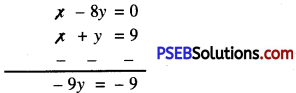y = 1
Substitute this value of y in (2), we get :
x – 8 × 1 = 0
or x = 8
Hence, required number = 10y + x
= 10 × 1 + 8 = 18.

(iv) Let, Meena received number of Rs. 50 notes = x
also, Meena received number of Rs. 100 notes = y
According to 1st condition,
x + y = 25 ……………(1)
According to 2nd condition,
50x + 100y = 2000
or x + 2y = 40 ………………(2)
Now, (2) – (1) gives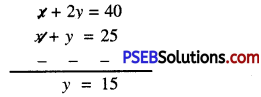Substitute this value of y in (1), we get:
x + 15 = 25
or x = 25 – 15 = 10
Hence, Meena received number of notes of
Rs. 50 and Rs. 100 are 10 and 15 respectively.(v) Let fixed charges for first three days = ₹ x
An additional charge for each day thereafter = ₹ y
In case of Saritha,
x + 4y = 27 …………..(1)
In case of Susy,
x + 2y = 21
Now, (1) – (2) gives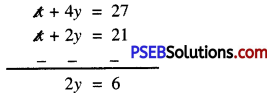or y = $$\frac{6}{2}$$ = 3
Substitute this value of y in (2), we get:
x + 2(3) = 21
or x + 6 = 21
or x = 21 – 6 = 15
Hence, fixed charges for first three days and an additional charge for each day thereafter ₹ 15 and ₹ 3.

## PSEB 10th Class English Book Solutions | PSEB 10th Class English Guide

Punjab State Board Syllabus PSEB 10th Class English Book Solutions Guide Pdf is part of PSEB Solutions for Class 10.

## PSEB 10th Class English Guide | English Guide for Class 10 PSEB

English Guide for Class 10 PSEB | PSEB 10th Class English Book Solutions

### PSEB 10th Class English Main Course Book Solutions

English Main Course Book Class 10 Solutions PSEB Prose

Class 10th English Main Course Book Solutions PSEB Poetry

### PSEB 10th Class English Literature Book Solutions

10th Class English Literature Book PSEB Supplementary Reader

### 10th Class PSEB English Grammar Book Solution

PSEB 10th Class English Book Grammar

PSEB 10th Class English Book Vocabulary

PSEB 10th Class English Book Reading Skills

PSEB 10th Class English Book Composition & Translation

English Grammar Notes is the one-stop-shop for students, beginners, and even experienced people to earn knowledge on all english related topics like Vocabulary, phrases, speeches, pronunciation, essay writings, tenses, etc.

### PSEB 10th Class English Structure of Question Paper

Class – X (English)

Time : 3 Mrs.

Theory: 80 marks
INA: 20 marks
(Listening and Speaking skill-based practical: 18 Marks
Book Bank: 2 Marks)
Total: 100 marks

 Q.No. Content Maximum Marks (80) 1. Section A : Reading Comprehension (a) Unseen Reading Comprehension (5 MCQs) (5Q × 1M = 5 Marks) (b) Unseen Picture/Poster Based Comprehension (5 MCQs) (5Q × 1M = 5 Marks) 2. Section B: Objective Type Questions It will consist of 8 objective-type questions carrying one mark each. Objective-type questions may include questions with one word to one-sentence answers. Fill in the blanks, True/False, or multiple choice type questions. English Main Course Book (4 Questions) English Supplementary Reader (4 Questions) (8Q × 1M = 8 Marks) 3. Section C: English Textbooks English Main Course Book: Prose (a) Short Answer Type Questions (3 out of 5) (3Q × 2M = 6 Marks) (b) Long Answer Type Questions (1 out of 2) (1Q × 3M = 3 Marks) 4. English Main Course Book: Poetry (a) Stanza (2 out of 3 questions) (2Q × 1.5M = 3 Marks) (b) Central Idea/Long Answer Type Questions (1 out of 2) (1Q × 3M = 3 Marks) 5. English Supplementary Reader (3 out of 5 questions) (3Q × 3M = 9 Marks) 6. Section D: Vocabulary Pair of Words, Idioms, Proverbs, One Word Substitution and Correct the Sentence (4Q × 1M = 4 Marks) 7. Section E: Translation, Grammar, and Composition (a) Translation from English to Punjabi/Hindi (3 out of 5) (3Q × 1M = 3 Marks) (b) Translation from Punjabi/Hindi to English (3 out of 5) (3Q × 1M = 3 Marks) 8. Grammar (Do as directed) (10Q × 1M = 10 Marks) 9. (a) Message/Advertisement/Note-making/Notice Writing (to attempt anyone out of the given two) (3 Marks) (b) Paragraph Writing (1 out of 2) given two) (4 Marks) (c) Letter Writing (1 out of 2) (6 Marks) 10. Marks for Good Handwriting (5 Marks)

Abbreviations used: q – question, m – marks

In Question 3(b), one long question will be asked from the first four chapters (‘The Happy Prince’, ‘Where Is Science Taking us?’, ‘Secret of Happiness’, and A Gift for Christmas’). The other question will be asked from the next four chapters (‘Some Glimpses of Ancient Indian Thought and Practices’, ‘The Home Coming’, ‘The Making of the Earth’ and ‘The Rule of the Road’).

Questions can be set from the entire prescribed syllabus. Only for questions No. 3, 4, and 5, questions will come from the given back exercises only. For the rest of the paper, it is not mandatory that the questions asked by the Paper-setter are from the back exercises only.

Listening and Speaking skill-based practical: 18 Marks

• Listening – 10 Marks
• Speaking – 08 Marks

For the listening test, students will be given a practice sheet containing 10 questions. They will answer all the 10 questions on the basis of an audio clip. Each question will carry 1 mark.

For the speaking test, students will speak 8 correct sentences on the basis of ‘picture and cue words’ provided in the practice sheet. Each correct sentence will carry 1 mark.

### PSEB 10th Class English Syllabus

• Reading Comprehension of unseen passage with five multiple-choice questions.
• Unseen Picture/Poster-based Comprehension with five multiple-choice questions.

2. English Main Course Book

1. The Happy Prince
2. Where Is Science Taking Us?
3. Secret of Happiness
5. Some Glimpses of Ancient Indian Thought and Practices
6. The Home-Coming
7. The Making of the Earth
8. The Rule of the Road

3. Poems

1. Character of a Happy Man
2. Death the Leveller
3. A Ballad of Sir Pertab Singh
4. Razia, the Tigress
5. Where the Mind is Without Fear

1. Bed Number – 29
2. Half a Rupee Worth
3. One Thousand Dollars
4. The Dying Detective
5. How Much Land Does a Man Need?

5. Vocabulary

• Pairs of Words Generally Confused – 1 to 40
• Idioms – 1 to 40
• One Word for Many – 1 to 40
• Common Errors – All
• Common Proverbs – 1 to 40

6. Grammar

• Use of Determiners, Prepositions, Modals, and Sentence Connectors
• Use and Kinds of Non-Pinites
• Simple, Complex, and Compound Sentences
• Voice
• Reported Speech
• Use of Tenses
• Punctuation

7. Writing Skills

• Notice and Note-making
• Messages
• Paragraphs
• Letters (Personal, Business & Official)

8. Translation from English to Punjabi/Hindi and Translation from Punjabi/Hindi to English.

## PSEB 10th Class Hindi Book Solutions | PSEB 10th Class Hindi Guide

Punjab State Board Syllabus PSEB 10th Class Hindi Book Solutions Guide Pdf is part of PSEB Solutions for Class 10.

## PSEB 10th Class Hindi Guide | Hindi Guide for Class 10 PSEB

Hindi Guide for Class 10 PSEB | PSEB 10th Class Hindi Book Solutions

कविता भाग

कहानी भाग

निबंध भाग

एकांकी भाग

PSEB 10th Class Hindi Book Vyakaran

## PSEB 11th Class Maths Book Solutions Guide in Punjabi English Medium

Punjab State Board Syllabus PSEB 11th Class Maths Book Solutions Guide Pdf in English Medium and Punjabi Medium are part of PSEB Solutions for Class 11.

## PSEB 11th Class Maths Guide | Maths Guide for Class 11 PSEB in English Medium

PSEB 11 Class Math Book Pdf Chapter 1 Sets

Punjab Board Maths Book Class 11 Solutions Chapter 2 Relations and Function

11th Class Math Book PSEB Chapter 3 Trigonometric Functions

PSEB Class 11 Maths Solutions Chapter 4 Principle of Mathematical Induction

PSEB Class 11 Maths Syllabus Chapter 6 Linear Inequalities

PSEB 11th Class Math Book Pdf Chapter 7 Permutations and Combinations

11th Class Maths Solutions PSEB Chapter 8 Binomial Theorem

PSEB 11th Class Maths Solutions Chapter 9 Sequences and Series

PSEB 11th Class Math Book Pdf Chapter 10 Straight Lines

PSEB Class 11 Maths Syllabus Chapter 11 Conic Sections

PSEB Class 11 Maths Book Pdf Download Chapter 12 Introduction to three Dimensional Geometry

PSEB Class 11 Maths Solutions Chapter 13 Limits and Derivatives

11th Class Math Book PSEB Chapter 14 Mathematical Reasoning

Punjab Board Maths Book Class 11 Solutions Chapter 15 Statistics

PSEB 11 Class Math Book Pdf Chapter 16 Probability

## PSEB 12th Class Sociology Notes in Punjabi English Medium

Punjab State Board Syllabus PSEB 12th Class Sociology Notes Pdf in English Medium and Punjabi Medium are part of PSEB Solutions for Class 12.

## PSEB 11th Class Physics Book Solutions Guide in Punjabi English Medium

Punjab State Board Syllabus PSEB 11th Class Physics Book Solutions Guide Pdf in English Medium and Punjabi Medium are part of PSEB Solutions for Class 11.

## PSEB 12th Class Physical Education Notes in Punjabi English Medium

Punjab State Board Syllabus PSEB 12th Class Physical Education Notes Pdf in English Medium and Punjabi Medium are part of PSEB Solutions for Class 12.

## PSEB 12th Class Physical Education Notes in English Medium

PSEB 12th Class Physical Education Practical